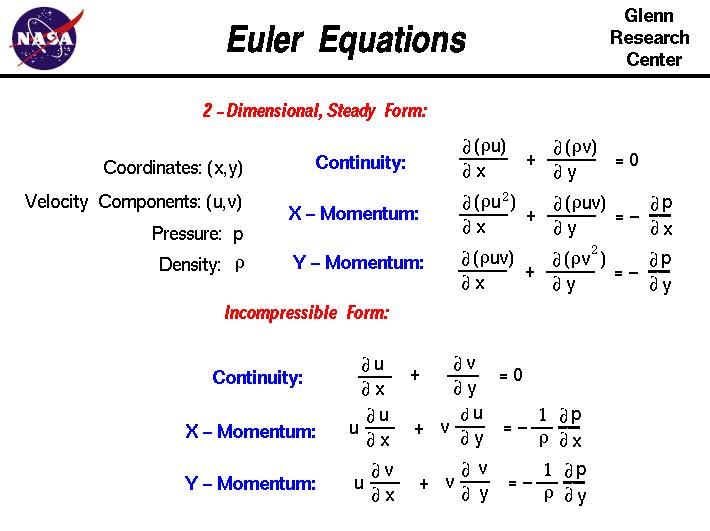A# fluid flowShown here are two versions of the Euler equations which describe how the velocity, pressure and density of a moving fluid are related. The equations are a set of coupled differential equations and they can be solved for a given flow problem by using methods from calculus. Though the equations appear to be very complex, they are actually simplifications of the more general Navier-Stokes equations of fluid dynamics. The Euler equations neglect the effects of the viscosity of the fluid which are included in the Navier-Stokes equations. A solution of the Euler equations is therefore only an approximation to a real fluids problem. Credit: NASA.

A fluid flow (both liquid and air) may be described in two different ways: the Lagrangian approach (named after the French mathematician Joseph Louis Lagrange), and the Eulerian approach (named after Leonhard Euler, a famous Swiss mathematician). In the Lagrangian approach, one particle is chosen and is followed as it moves through space with time. The line traced out by that one particle is called a particle pathline. An example is a transmitting ocean buoy that observes a set path over regular intervals over a period of time. The path observed is the particle pathline.

A Eulerian approach is used to obtain a clearer idea of the airflow at one particular instant. One can look at a "photograph" of the flow of, for instance, surface ocean currents at a particular fixed time. The entire flow field is easily visualized. The lines comprising this flow field are called streamlines (see streamlining).

Thus, a pathline refers to the trace of a single particle in time and space whereas a streamline presents the line of motion of many particles at a fixed time. The question of whether particle pathlines and streamlines are ever the same is considered next.

Of basic importance in understanding fluid movements about an object is the concept of a steady flow. On a windy day a person calls the wind steady if, from where she stands, it blows constantly from the same direction at a constant speed. If, however, the speed or direction changes, the wind is "gusty" or unsteady. In a similar manner the flow of a fluid (both liquid and air) about an object is steady if its velocity (speed and direction) at each point in the flow remains constant – this does not necessarily require that the velocity be the same at all points in the fluid.

This means that for unsteady flows, particle pathlines (the Lagrangian point of view) and streamlines (the Eulerian approach) are not equivalent. For a steady flow, however, a particle pathline and streamline are equivalent, and the Lagrangian point of view is the same as the Eulerian approach for flow visualization.

As well as steady or unsteady, fluid flow can be rotational or irrotational. If the elements of fluid at each point in the flow have no net angular (spin) velocity about the points, the fluid flow is said to be irrotational. One can imagine a small paddle wheel immersed in a moving fluid. If the wheel translates (or moves) without rotating, the motion is irrotational. If the wheel rotates in a flow, the flow is rotational.

According to a theorem of Hermann von Helmholtz, a German physicist who contributed much to theoretical aerodynamics, assuming zero viscosity, if a fluid flow is initially irrotational, it remains irrotational. In real life, viscosity effects are limited to a small region near the surface of the airfoil and in its wake. Most of the flow may still be treated as irrotational.

A simplifying argument often used to aid in understanding basic ideas about fluid flow is that of a one-dimensional fluid flow. Flows may be considered one-dimensional where the flow parameters (for example: density, velocity, temperature, pressure) vary as a function of one spatial variable (for example, length) and variations in the other two spatial dimensions (i.e., y and z) are negligible by comparison.

To make study of fluids somewhat easier, simplifying assumptions about fluids are made. The first is that fluid is considered to be inviscid (no viscosity); the second is that it is incompressible. Further, the flow is considered steady and one-dimensional. Fluids with these characteristics are said to be ideal fluids or perfect fluids. Once solutions of problems relating to the lift and drag of ideal fluids, or the inviscid flow, have been made, a solution of the viscous flow in the thin boundary layer allows the effects of skin friction drag to be calculated.

## Basic equations of fluid flow

The velocity, pressure, and density of a moving fluid are related by three basic equations: the equation of continuity, which relates the amount of fluid flowing into a given space with the amount flowing out of that space; Euler's equation of motion, which shows how the velocity of the fluid changes with time at a given point in space; and the adiabatic equation, which describes the exchange of heat between different parts of the fluid. In incompressible fluid flow, these equations take on a particularly simple form.

The equations for realistic fluid are so complicated that complete solutions to most problems do not exist; numerical solutions to most problems must be attempted by computer techniques.